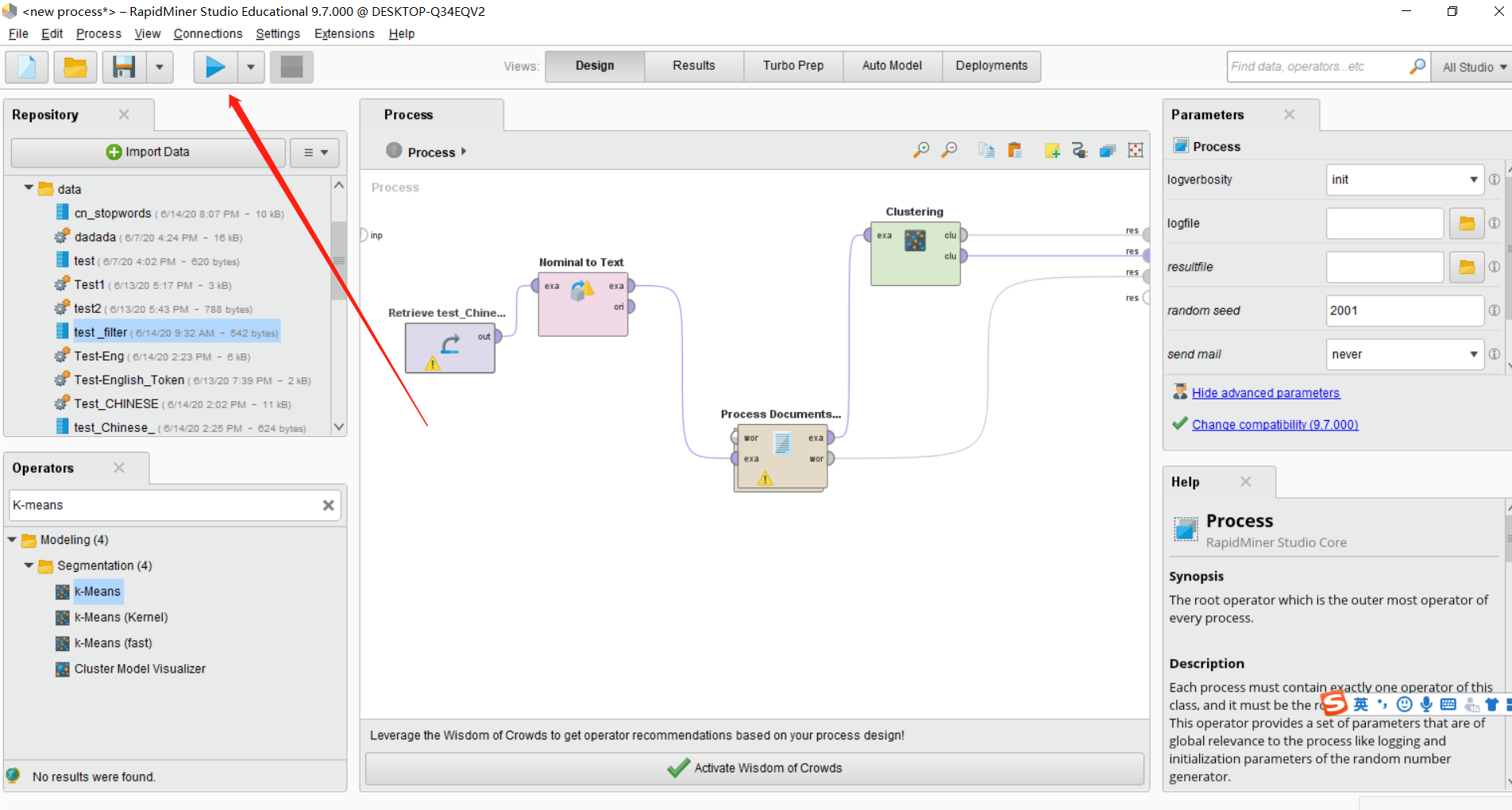Rapidminer 利用python插件替代原有只支持英文的功能进行中文分词&&去除停用词

# Rapidminer 中文分词&&去除停用词

## First: 打开Rapidminer新建项目## Second: 导入数据集&&导入停用词列表`File`，点击`Import Data`选项。会出现下面画面：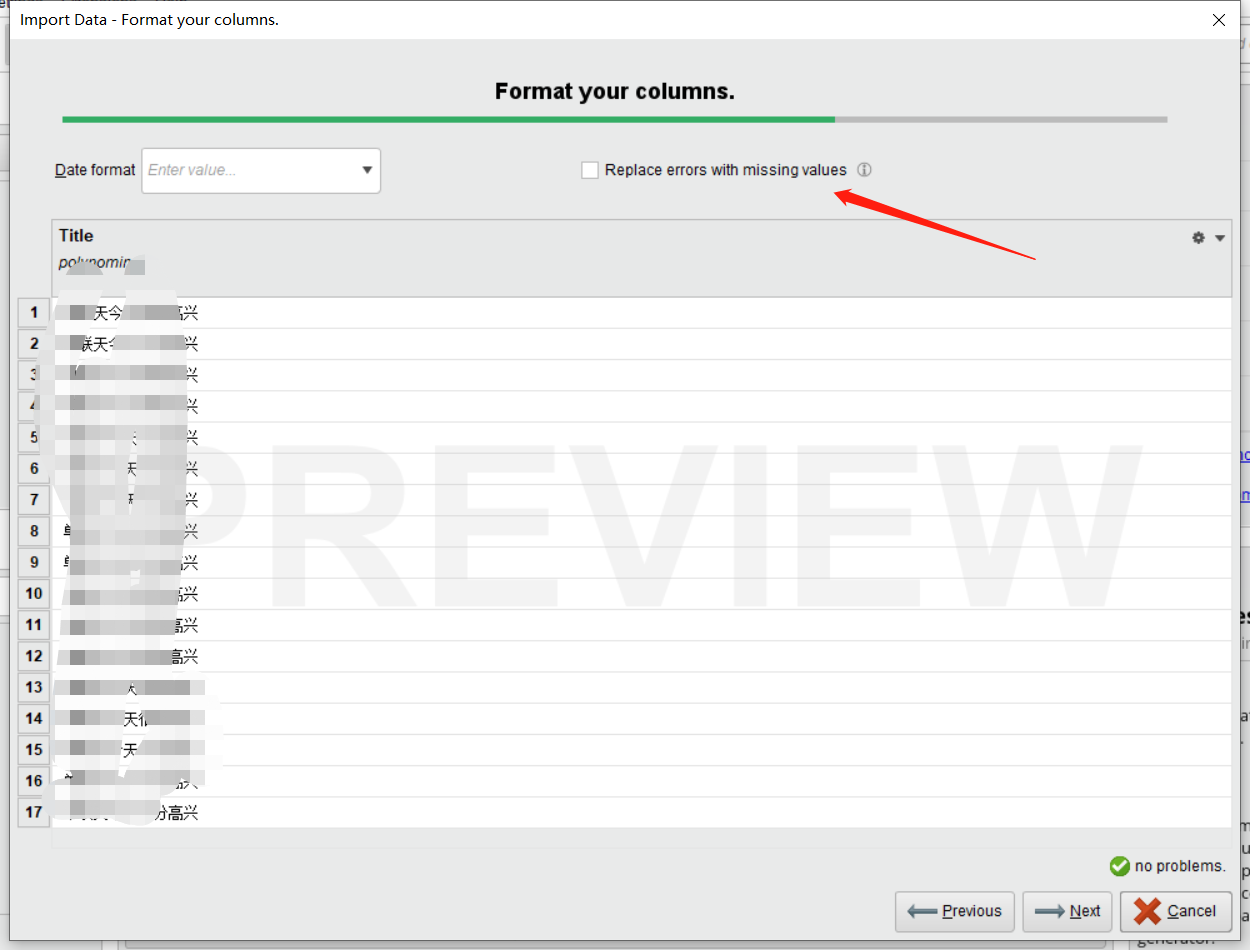## Third: 构建整个流程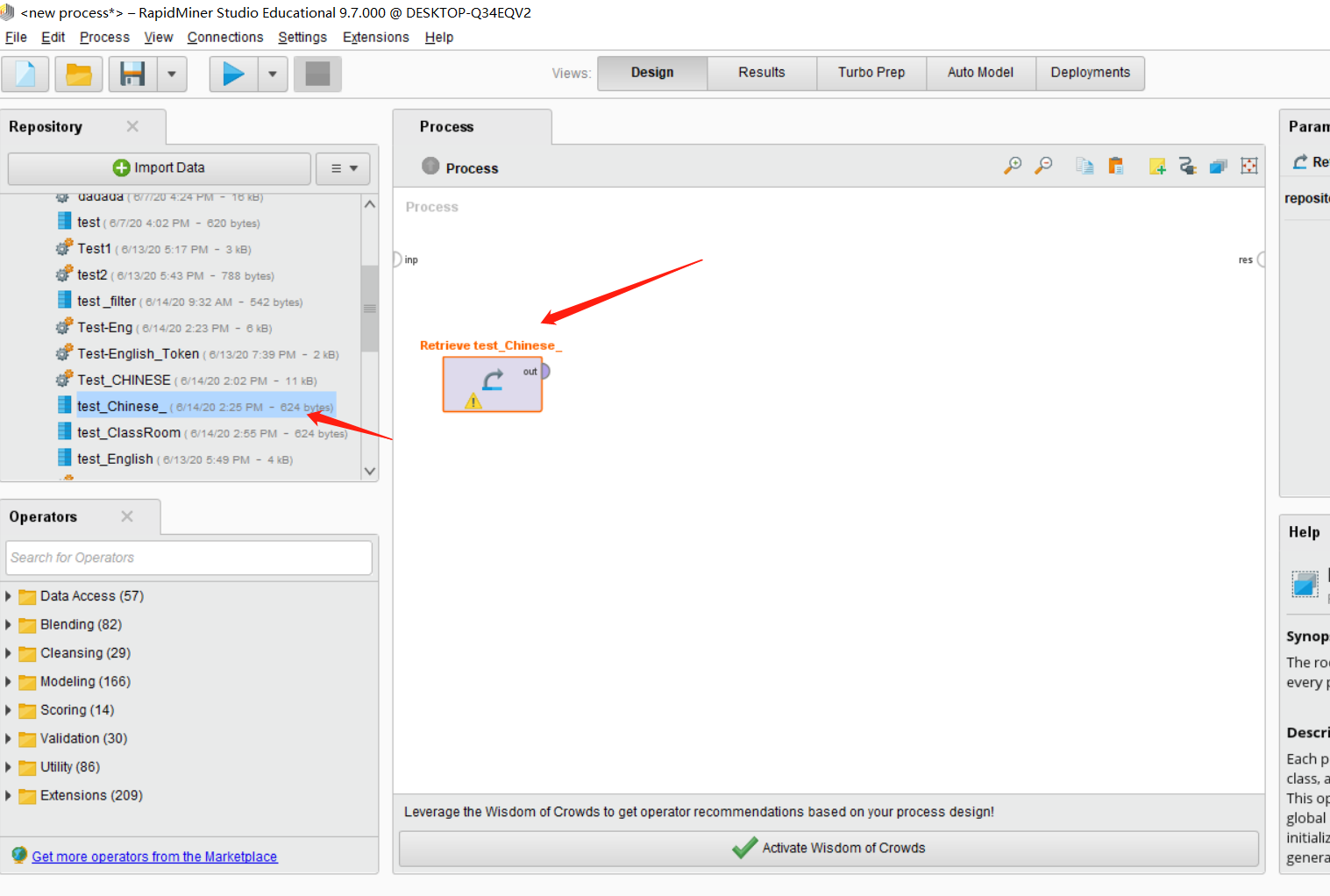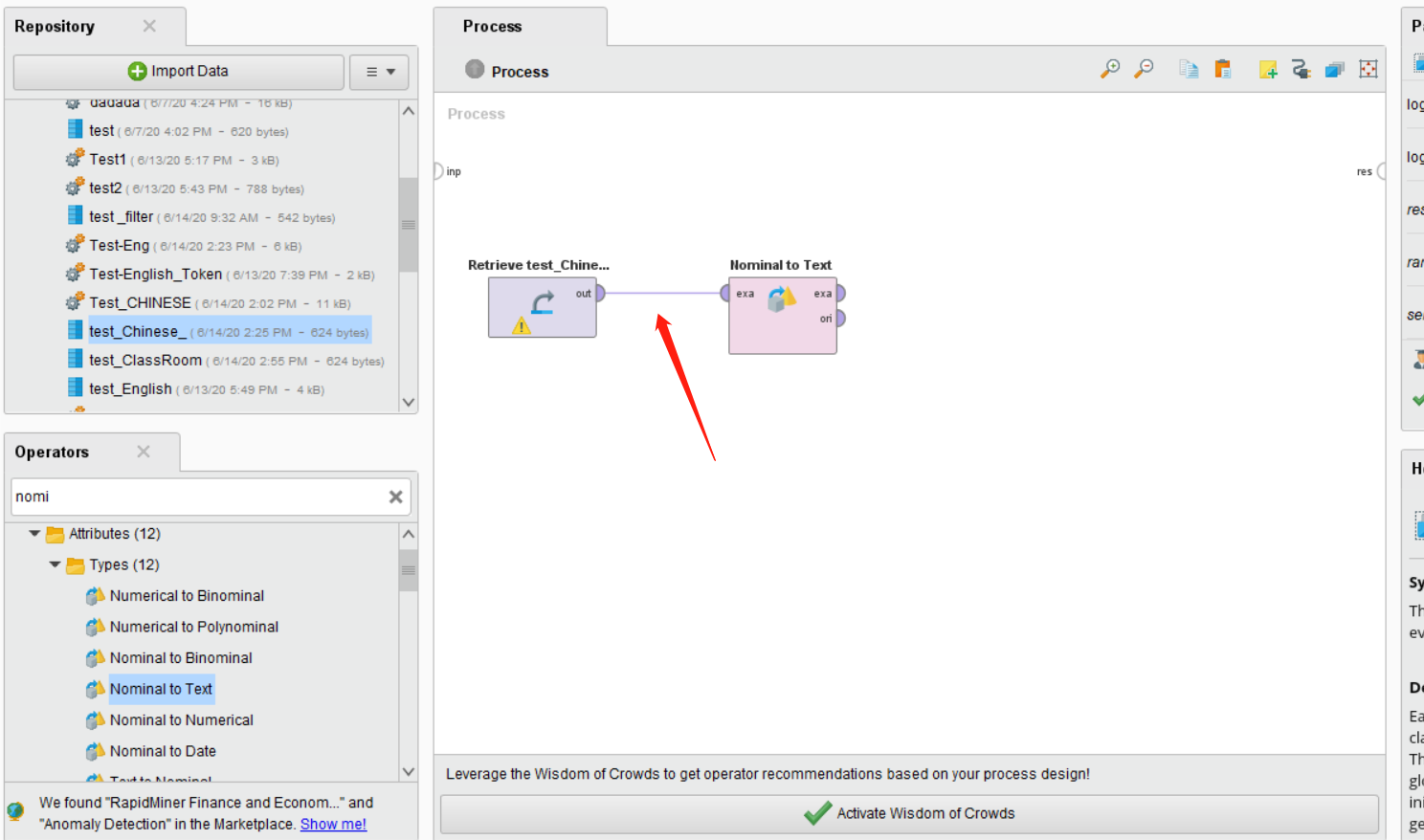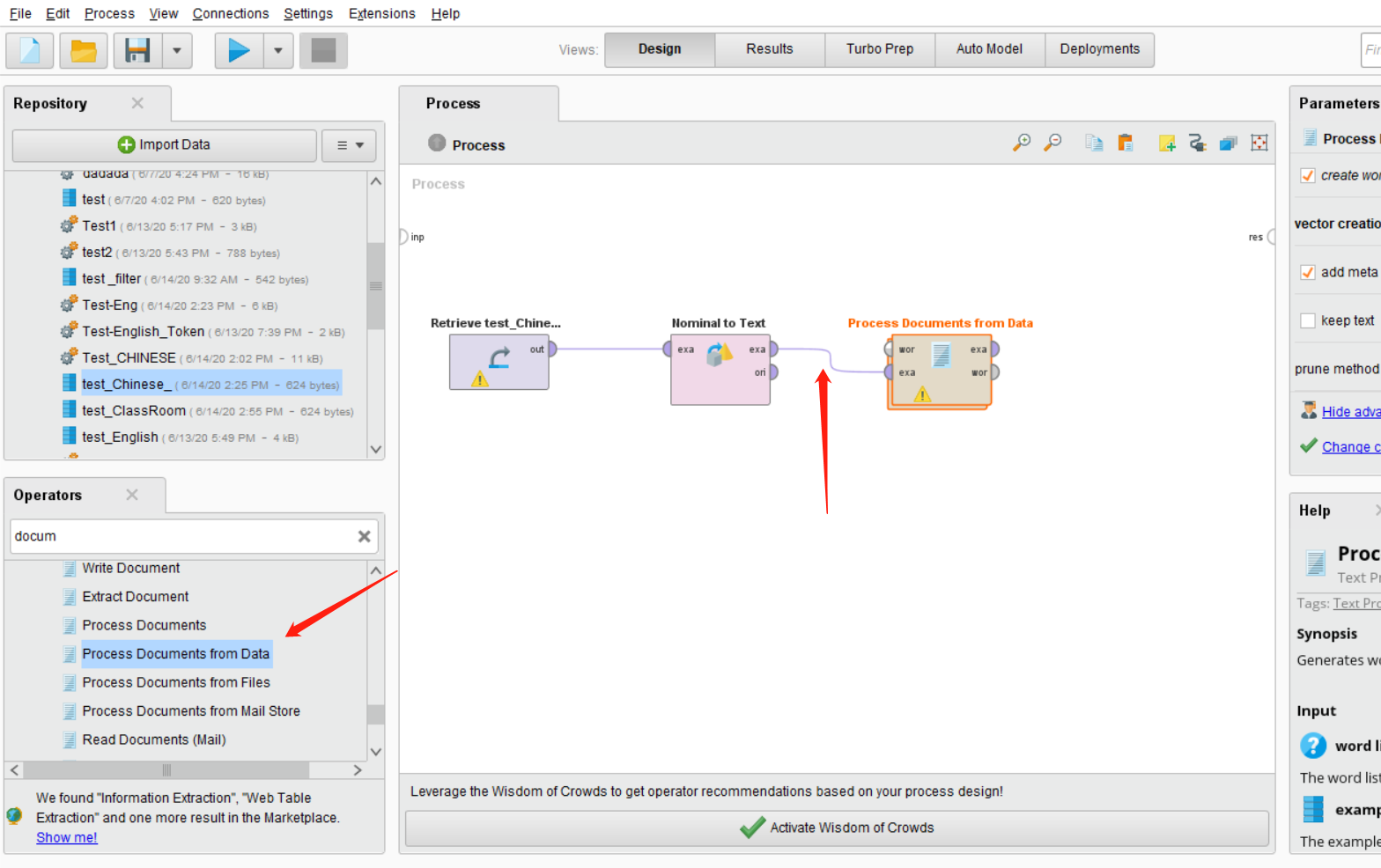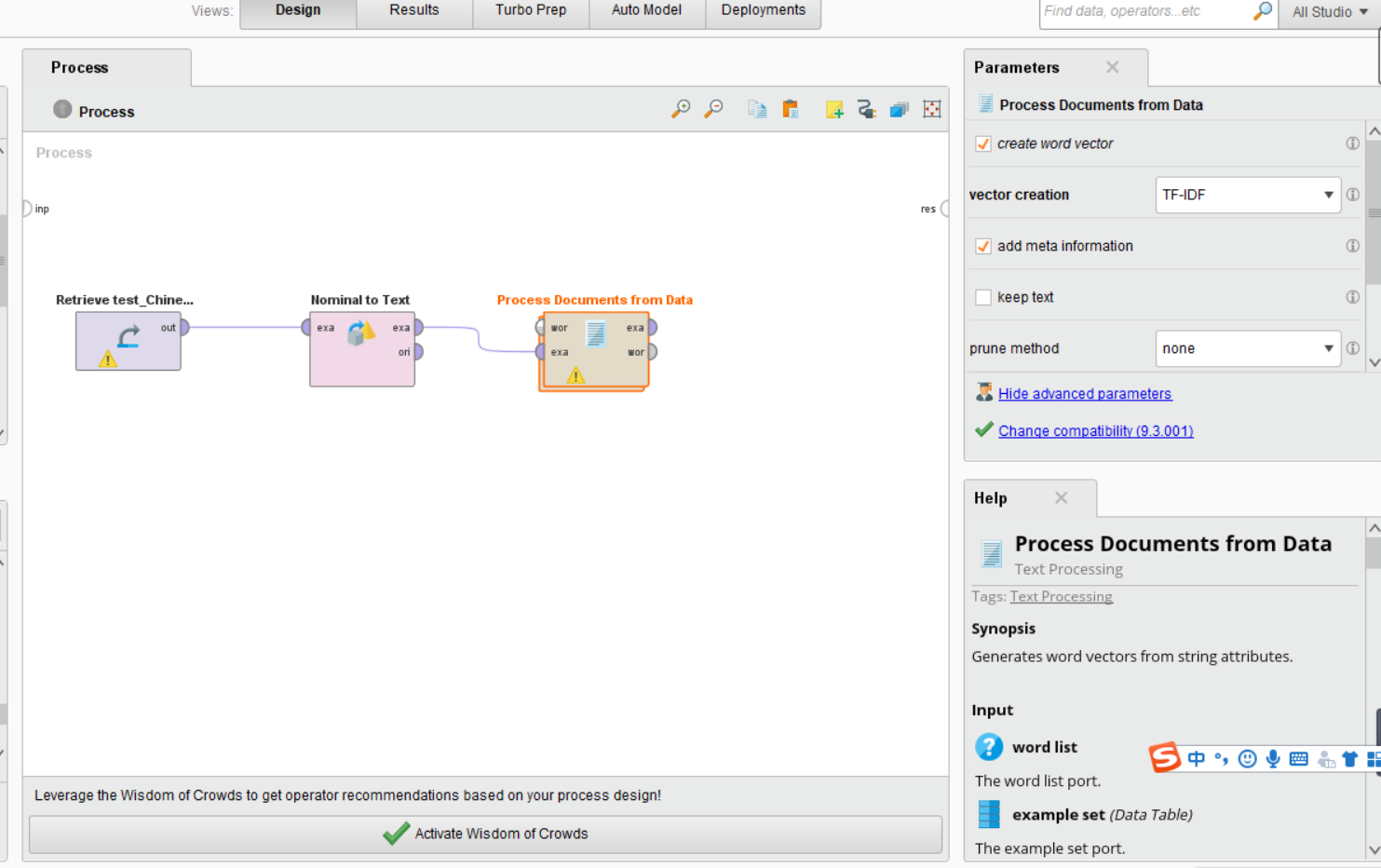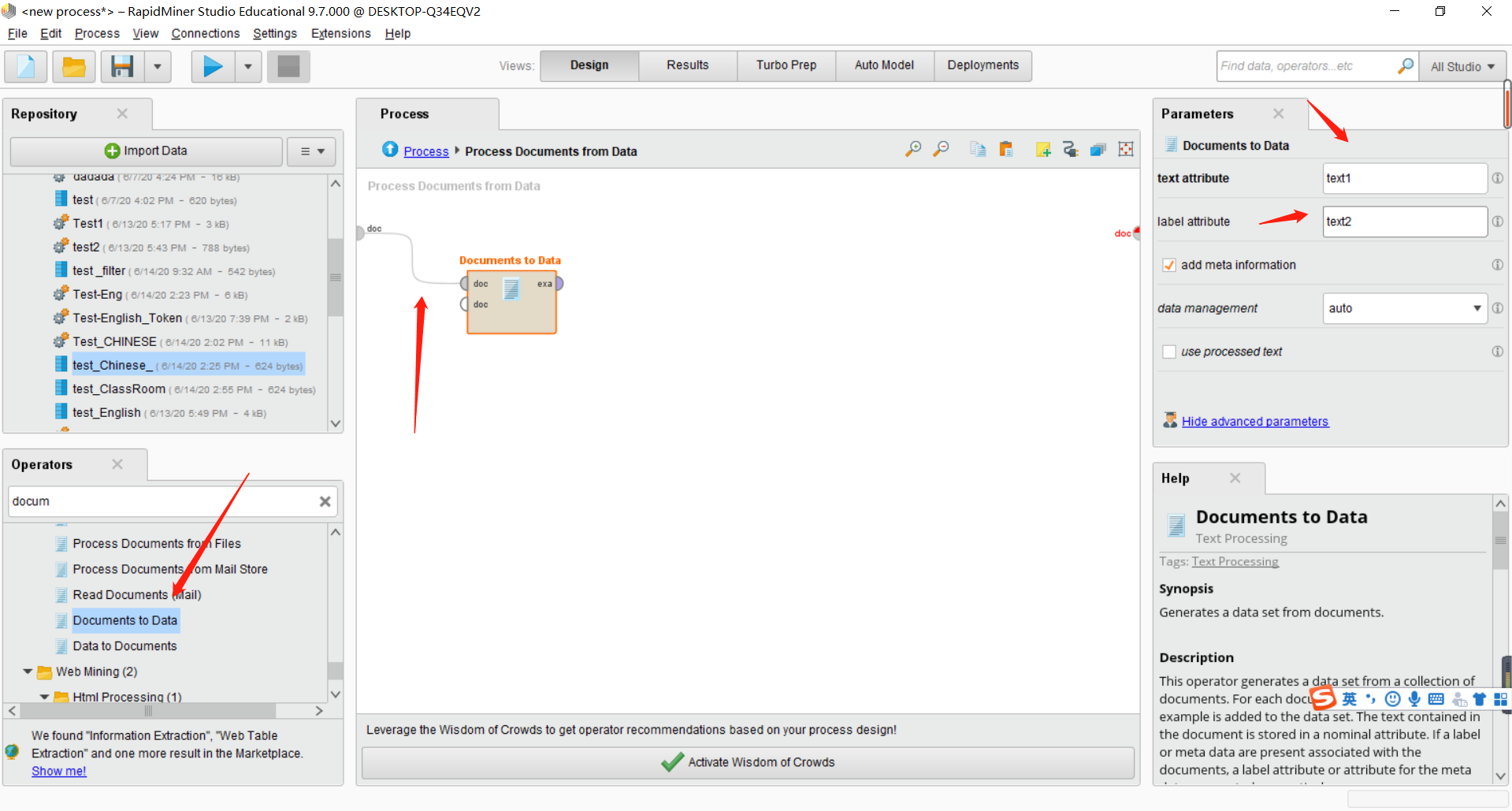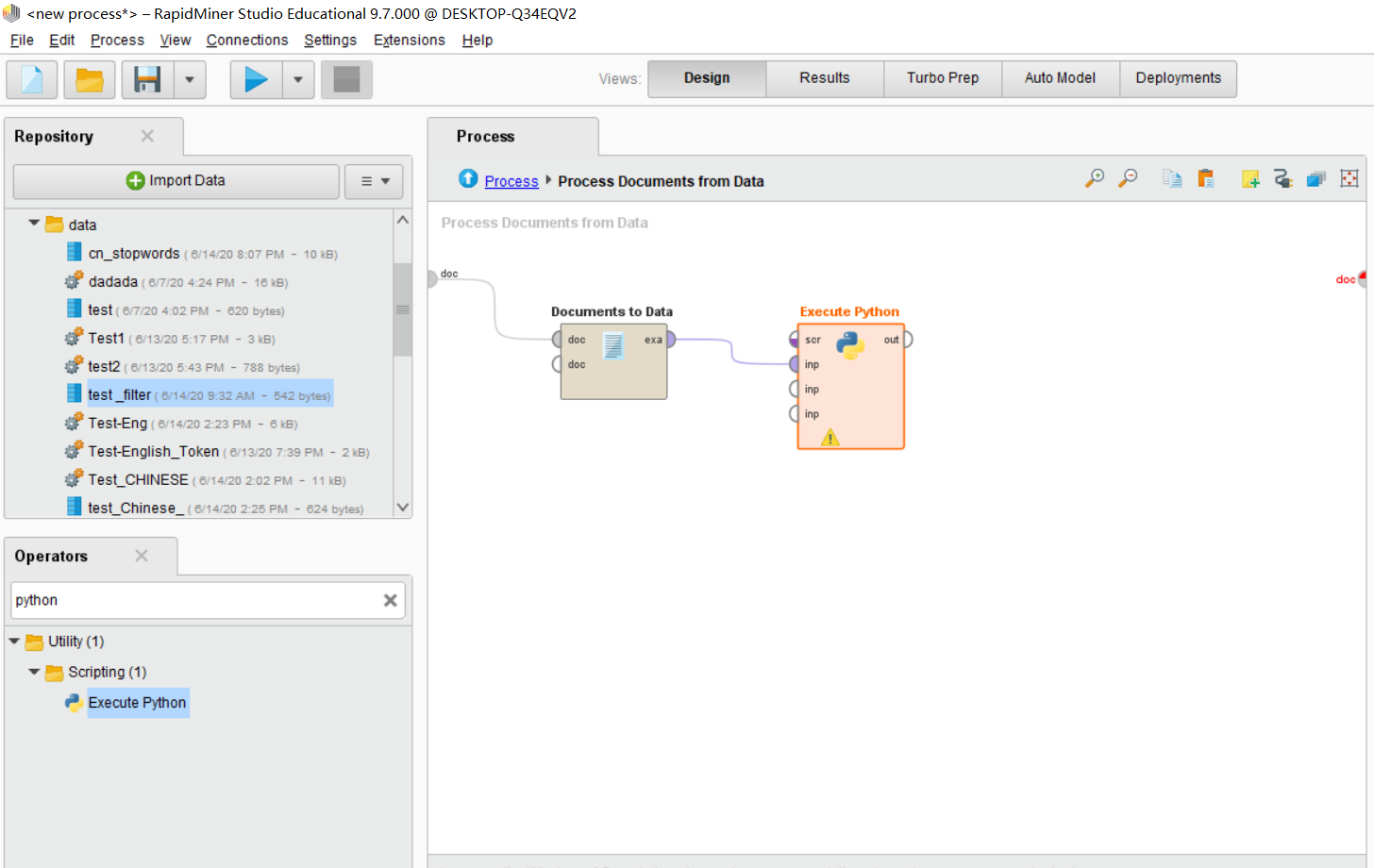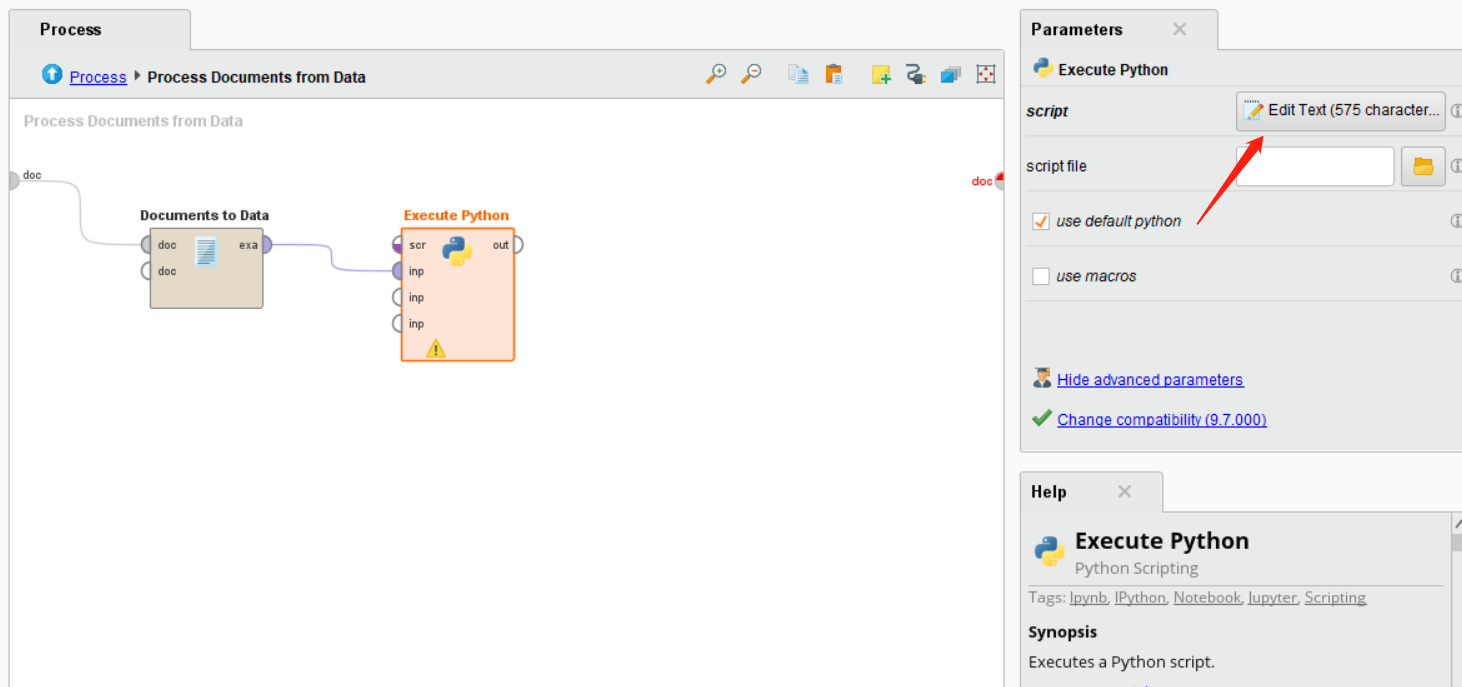``````import pandas as pd
import jieba
import jieba.analyse
#里面很多无关紧要的代码，但是可以测试用。
# rm_main is a mandatory function,
# the number of arguments has to be the number of input ports (can be none)
def rm_main(data):
# output can be found in Log View
segments = [] #功能留用
framedata = []
for index, row in data.iterrows():
content=row
words = jieba.cut(content)
splitedStr = ''
for word in words:
#停用词判断，如果当前的关键词不在停用词库中才进行记录
segments.append({'word':word, 'count':1})#可有可无
splitedStr += word + ' '
framedata.append({'splited word':splitedStr})
Sgdata = pd.DataFrame(framedata)
list1 = []
for i in range(len(Sgdata)):
lis = Sgdata.iloc[i].strip().split(' ')
list1.append(lis)
# connect 2 output ports to see the results
Sgdata = pd.DataFrame(list1)
return Sgdata``````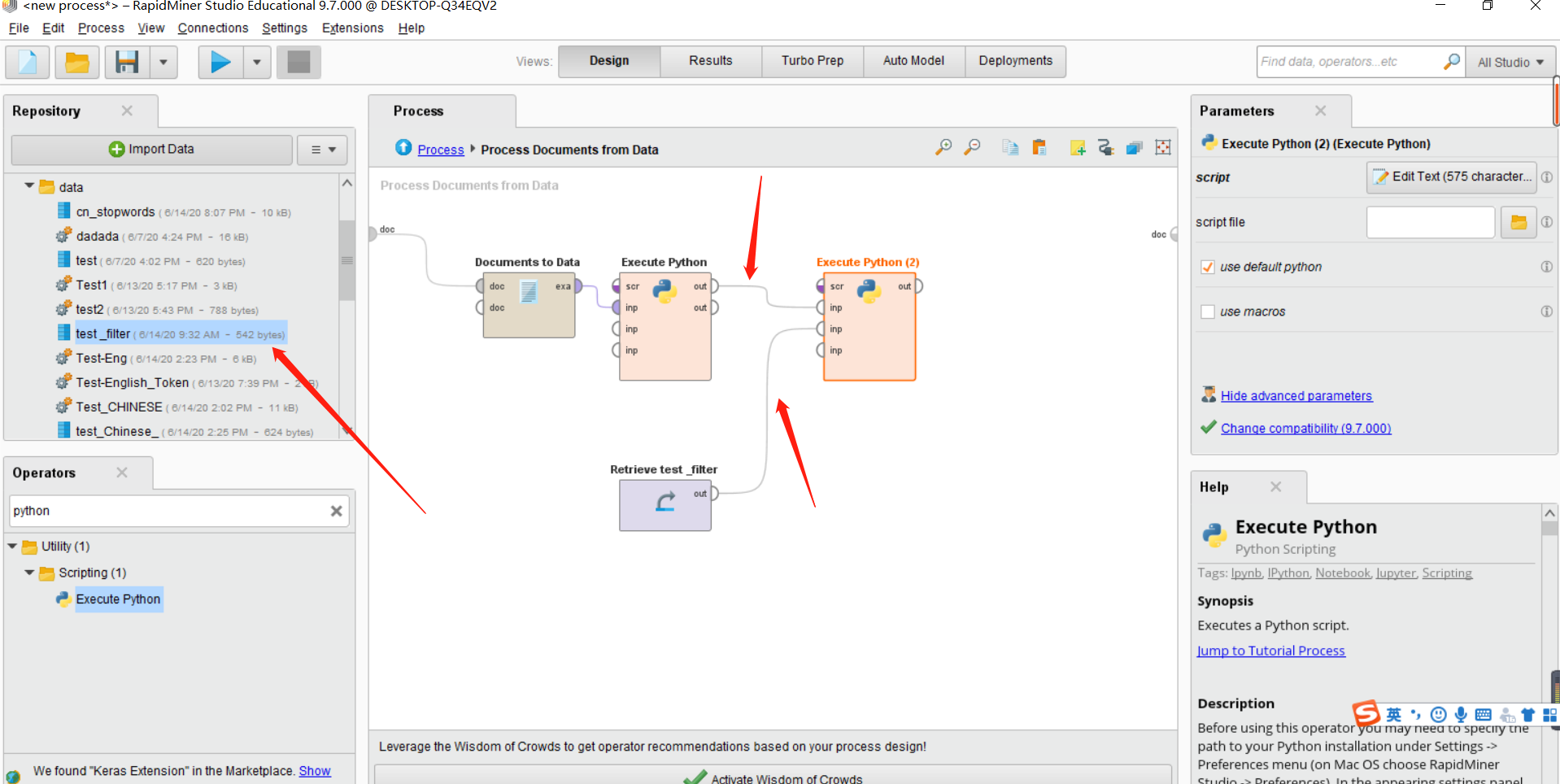``````import pandas as pd
import numpy as np
def rm_main(data,data1):
# output can be found in Log View
listdata = np.array(data1)
stopwords = listdata.tolist()
segments = []  # 功能留用
col=k=data.shape
My_content=[]
framedata = []
j = 0  # 记录列数
for index, row in data.iterrows():
My_content.append([])
for i in range(0,k):
content=row[i]
My_content[j].append(content)
splitedStr = ''
for l in range(0,col):
stop = []
if My_content[j][l]!=None:
stop.append(My_content[j][l])
if stop not in stopwords:
# 记录全局分词
segments.append({'word': My_content[j][l], 'count': 1})
splitedStr += My_content[j][l] + ' '
j = j + 1
framedata.append({'splited word': splitedStr})
Sgdata = pd.DataFrame(framedata)
list1 = []
for i in range(len(Sgdata)):
lis = Sgdata.iloc[i].strip().split(' ')
list1.append(lis)
# connect 2 output ports to see the results
Sgdata = pd.DataFrame(list1)
return Sgdata``````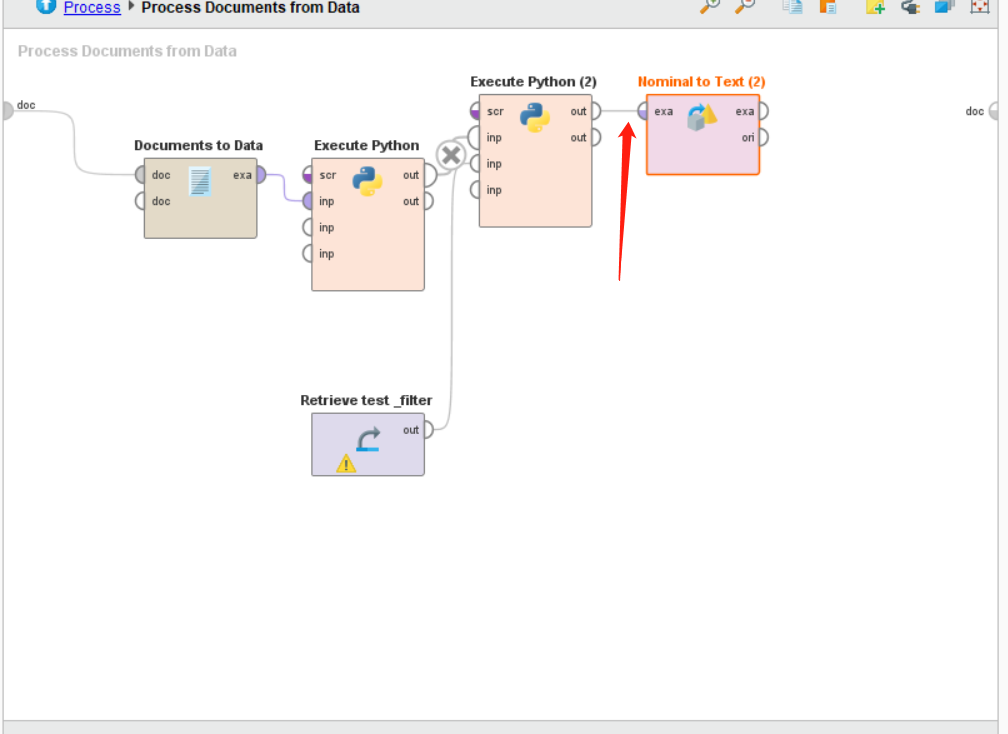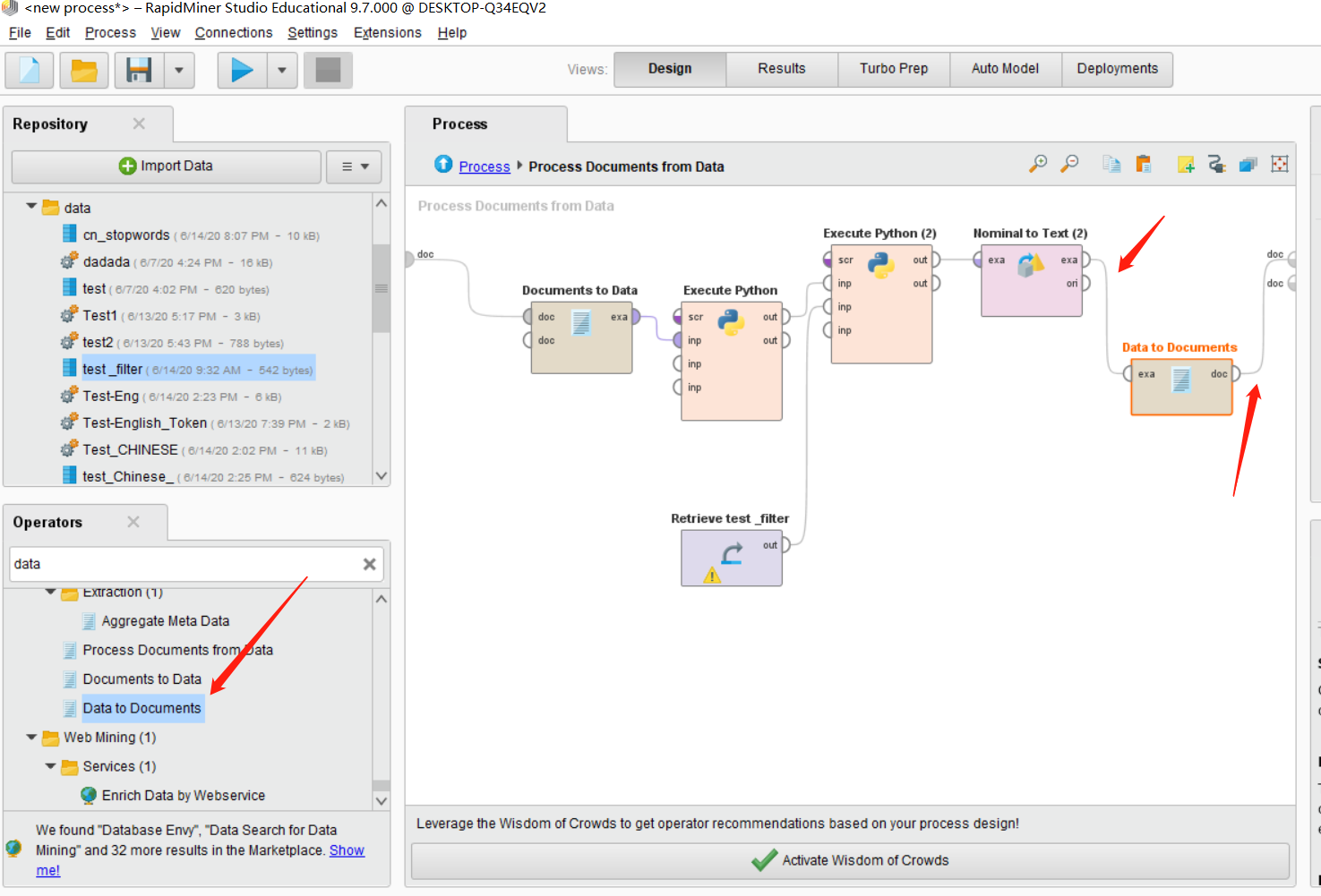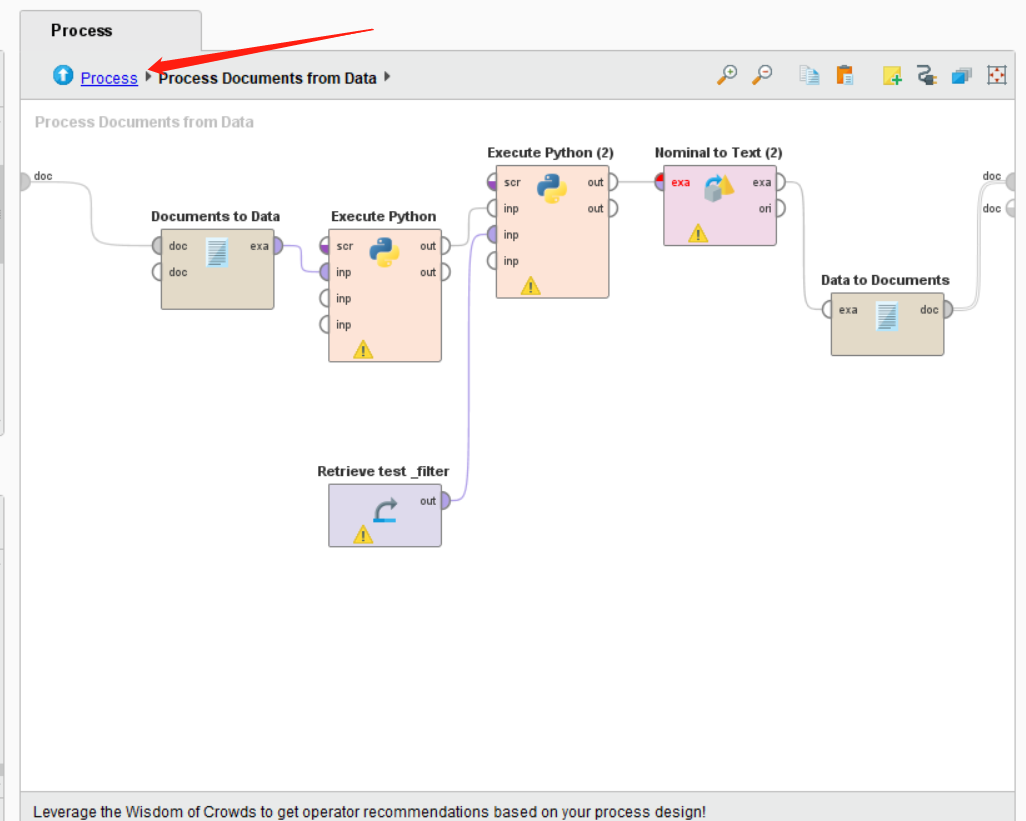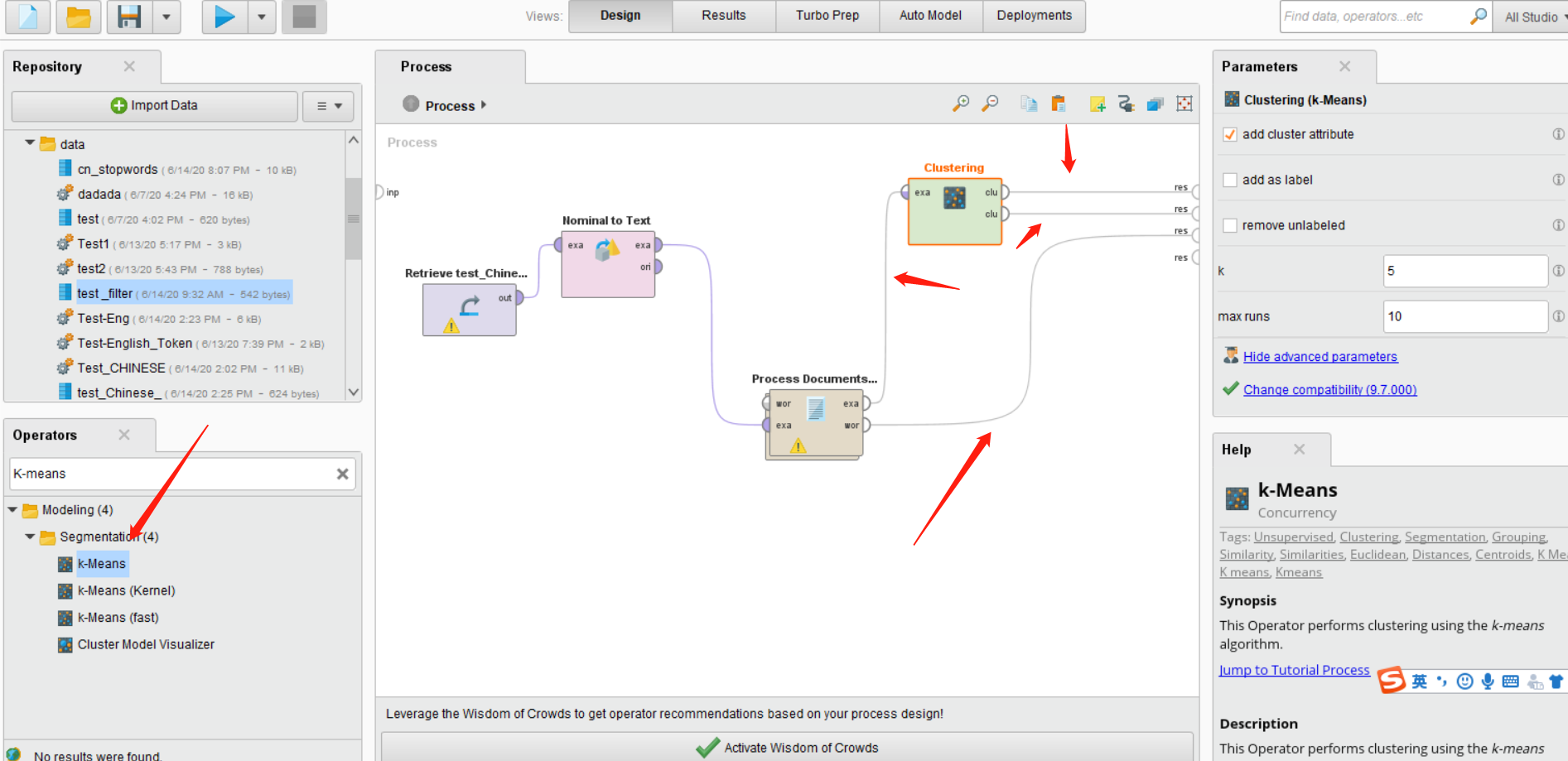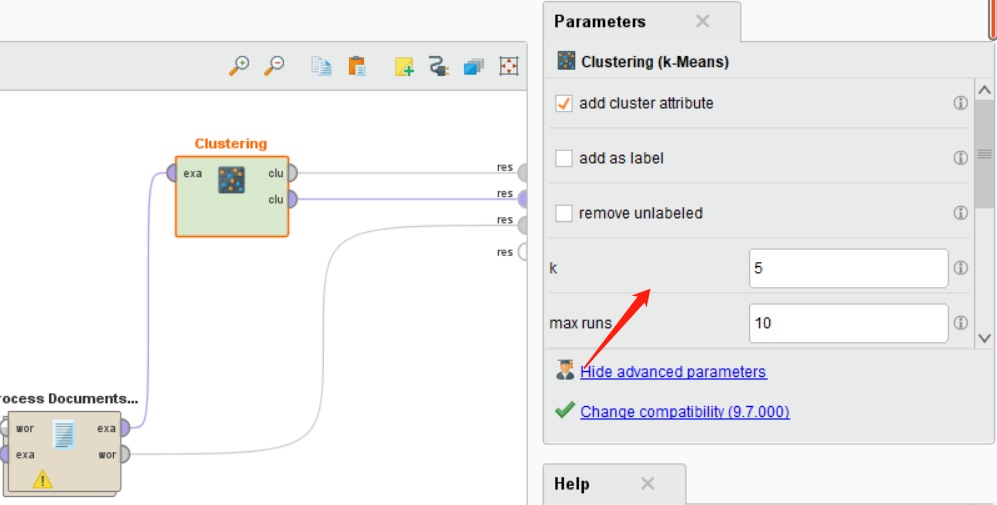## Fourth: 运行整个流程